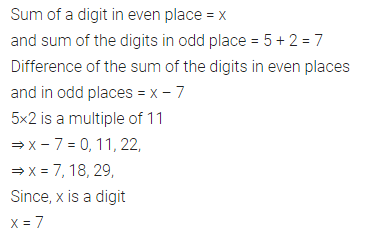# Selina Concise Mathematics Class 8 ICSE Solutions Chapter 5 Playing with Number

## Selina Concise Mathematics Class 8 ICSE Solutions Chapter 5 Playing with Number

Selina Publishers Concise Mathematics Class 8 ICSE Solutions Chapter 5 Playing with Number

### Playing with Number Exercise 5A – Selina Concise Mathematics Class 8 ICSE Solutions

Question 1.
Write the quotient when the sum of 73 and 37 is divided by
(i) 11
(ii) 10
Solution: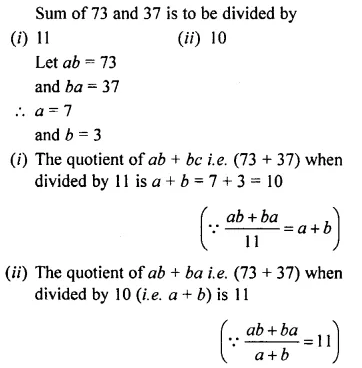Question 2.
Write the quotient when the sum of 94 and 49 is divided by
(i) 11
(ii) 13
Solution:Question 3.
Find the quotient when 73 – 37 is divided by
(i) 9
(ii) 4
Solution: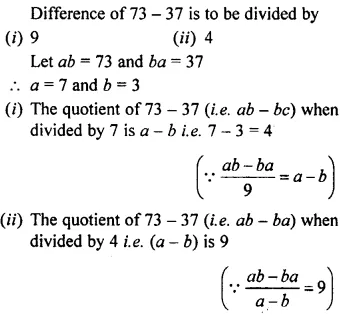Question 4.
Find the quotient when 94 – 49 is divided by
(i) 9
(ii) 5
Solution:Question 5.
Show that 527 + 752 + 275 is exactly divisible by 14.
Solution:Question 6.
If a = 6, show that abc = bac.
Solution:Question 7.
If a > c; show that abc – cba = 99(a – c).
Solution:Question 8.
If c > a; show that cba – abc = 99(c – a).
Solution:Question 9.
If a = c, show that cba – abc = 0.
Solution: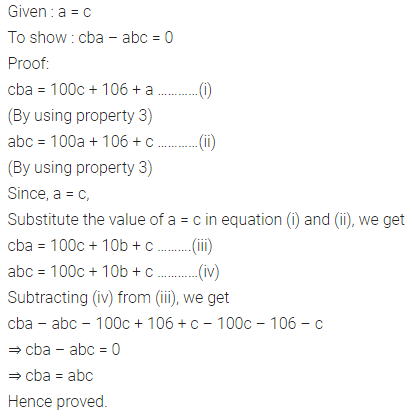Question 10.
Show that 954 – 459 is exactly divisible by 99.
Solution:### Playing with Number Exercise 5B – Selina Concise Mathematics Class 8 ICSE Solutions

Question 1.Solution: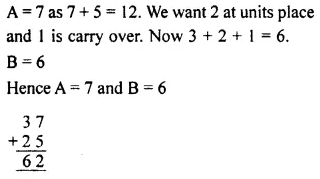Question 2.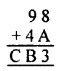Solution: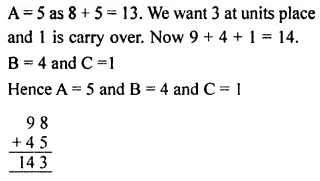Question 3.Solution:Question 4.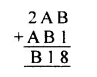Solution: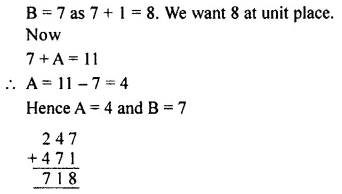Question 5.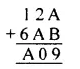Solution: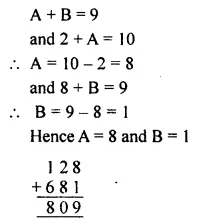Question 6.Solution: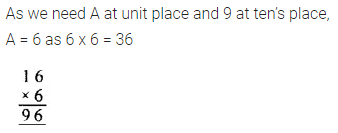Question 7.Solution: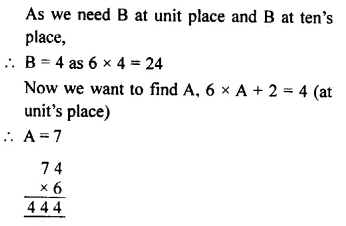Question 8.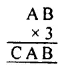Solution: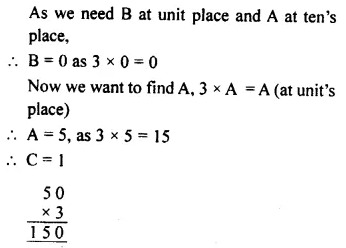Question 9.Solution:Question 10.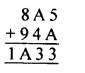Solution: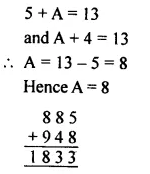Question 11.Solution: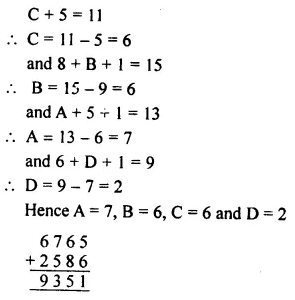### Playing with Number Exercise 5C – Selina Concise Mathematics Class 8 ICSE Solutions

Question 1.
Find which of the following nutpbers are divisible by 2:
(i) 192
(ii) 1660
(iii) 1101
(iv) 2079
Solution: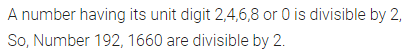Question 2.
Find which of the following numbers are divisible by 3:
(i) 261
(ii) 111
(iii) 6657
(iv) 2574
Solution: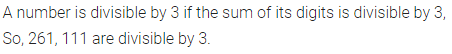Question 3.
Find which of the following numbers are divisible by 4:
(i) 360
(ii) 3180
(iii) 5348
(iv) 7756
Solution: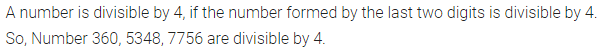Question 4.
Find which of the following numbers are divisible by 5 :
(i) 3250
(ii) 5557
(iii) 39255
(iv) 8204
Solution: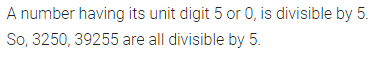Question 5.
Find which of the following numbers are divisible by 10:
(i) 5100
(ii) 4612
(iii) 3400
(iv) 8399
Solution:Question 6.
Which of the following numbers are divisible by 11 :
(i) 2563
(ii) 8307
(iii) 95635
Solution:### Playing with Number Exercise 5D – Selina Concise Mathematics Class 8 ICSE Solutions

For what value of digit x, is :
Question 1.
1×5 divisible by 3?
Solution:Question 2.
31×5 divisible by 3?
Solution: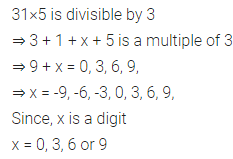Question 3.
28×6 a multiple of 3?
Solution: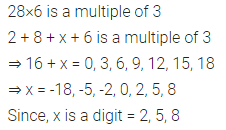Question 4.
24x divisible by 6 ?
Solution:Question 5.
3×26 a multiple of 6?
Solution:Question 6.
42×8 divisible by 4?
Solution: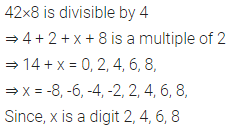Question 7.
9142x a multiple of 4?
Solution: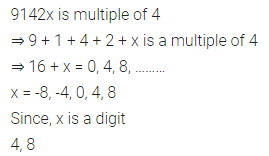Question 8.
7×34 divisible by 9?
Solution: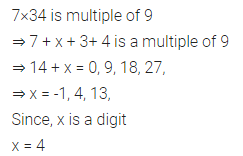Question 9.
5×555 a multiple of 9 ?
Solution: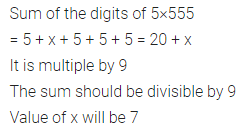Question 10.
3×2 divisible by 11?
Solution: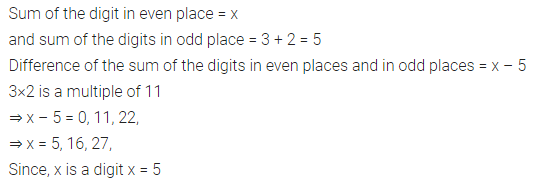Question 11.
5×2 a multiple of 11?
Solution: